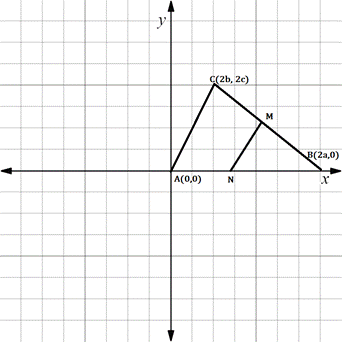Chapter 10.CT, Problem 20CTElementary Geometry For College St...

7th Edition
Alexander + 2 others
ISBN: 9781337614085

Solutions

Chapter
SectionElementary Geometry For College St...

7th Edition
Alexander + 2 others
ISBN: 9781337614085
Textbook Problem

Use the drawing provided for the proof of the theorem “The line segment that joins the midpoints of two sides of a triangle is parallel to the third side of the triangle.”Complete this Proof:Proof: Given △ A B C with vertices as shown, let M and n name the midpoints of sides C B - and A B - , respectively.

To determine

To prove:

The given theorem “The line segment that joins the midpoints of two sides of a triangle is parallel to the third side of the triangle”.

Explanation

The given figure is shown below.

We have to prove the given theorem.

Let A0, 0, B2a, 0 and C2b, 2c be the vertices of the triangle.

Let M be the midpoint of the line segment CB-.

Let N be the midpoint of the line segment AB-.

The line segment that joins the midpoints is MN.

We have to prove that MN- is parallel to AC-

That is, slope of MN- is equal to slope of AC-.

Find the vertices of M.

Since, M lies midway between B and C.

Find the midpoint of -

This is the coordinate of M.

x1,y1=B2a, 0

x2,y2=C2b, 2c

Formula for midpoint of the line segment joining two points.

M=x1+x22, y1+y22

Substituting the x and y co-ordinates.

M=2a+2b2, 0+2c2

M=2a+b2, 2c2

Cancelling 2,

M=a+b, c

Find the vertices of N.

Since, N lies midway between A and B.

Find the midpoint of AB-.

This is the coordinate of N.

x1,y1=A0, 0

x2,y2=B2a, 0

Substituting the x and y co-ordinates.

N=0+2a2, 0+02

N=2a2, 02

Cancelling 2,

N=a, 0

Now, prove that MN-AC-

Still sussing out bartleby?

Check out a sample textbook solution.

See a sample solution

The Solution to Your Study Problems

Bartleby provides explanations to thousands of textbook problems written by our experts, many with advanced degrees!

Get Started

In Exercises 99-106, factor out the greatest common factor from each expression. 103. ex xex

Applied Calculus for the Managerial, Life, and Social Sciences: A Brief Approach

Simplify the expressions in Exercises 97106. (xy)1/3(yx)2/3

Finite Mathematics and Applied Calculus (MindTap Course List)

Find the linearization L(x) of the function at n. 2. f(x) = sin x, a = /6

Single Variable Calculus: Early Transcendentals, Volume I

For , f(x) = 0 2 3 f(3) does not exist

Study Guide for Stewart's Multivariable Calculus, 8th

limx5x225x29x+20= a) 1 b) 10 c) d) does not exist

Study Guide for Stewart's Single Variable Calculus: Early Transcendentals, 8th